# The Difference in the Wall Thickness of the Helicopter Structure Are Made of Composite Materials with Another Made of Steel

The Difference in the Wall Thickness of the Helicopter Structure Are Made of Composite Materials with Another Made of Steel
Mechanical Technology Department, Technical Institute, Northern Technical University, Mosul 41000, Iraq

Power Mechanical Techniques Eng. Department, Tech. Engineering College, Northern Technical University, Mosul 41000, Iraq

Technical Engineering College, Northern Technical University, Mosul 41000, Iraq

Corresponding Author Email:
Page:
313-324
|
DOI:
https://doi.org/10.18280/mmep.090204
4 February 2022
|
Accepted:
6 April 2022
|
Published:
28 April 2022
| Citation

OPEN ACCESS

Abstract:

Weight is of great importance in the aircraft industry. Aircraft are made of aluminum alloys that are susceptible to heat treatment, because they are light in weight and are metal strong enough for the dynamic designed loads they can face, but there are other reasons for obtaining alternative materials, and these materials are composite materials that it is lighter in weight than aircraft made of aluminum, firstly, and secondly, it can be formed into attractive shapes, eliminating welding and rivets, and thirdly, it can be formed into aerodynamic shapes. This work is based on designing a three-dimensional model consisting of aluminum alloy (AA-6061-T6) of the structure helicopter and then comparing it with five other models of different metal and composite materials to obtain a structure that has the least weight among these models. The results indicate that the best model with the lowest weight is the fourth model consisting of carbon fiber, proportions and weight of a square meter and a thickness of (28 mm) than the weight of the first model consisting of aluminum and weighing (81 kg), it was less than (22.7%). Then the fifth model, which consisted of an outer layer of aluminum with a thickness of five millimeters and another inner layer of aluminum of the same thickness, and between the inner and outer layer eighteen layers of carbon fiber, where the percentage of decrease in it compared to the first model by up to (19.2%), and worse a model in terms of weight is the second model was made of steel, which has a weight that is almost twice the weight of the first model.

Keywords:

steel, helicopter, carbon fiber, fiber glass, composite materials, failure, structure

1. Introduction

In this paper, Sex three-dimensional mathematical models will be created, consisting of different materials (aluminum, steel, fiber glass, carbon fiber, aluminum with carbon fiber, steel with carbon fiber). In order to obtain the best structure for a helicopter that is light in weight and that resists missiles under the influence of penetration pressure and high temperature, using the ANSYS 15.0 program.

2. Materials and Model Analysis

Six three-dimensional models were created by fixing the width and height of the aircraft, where the largest dimensions were (L = 15.56 * h = 5.23 m) respectively shown in Figure 1 and by changing the thickness of the helicopter structure to obtain the same specifications in the five models and take thickness of first model (Aluminum model (t = 0.03 m)) in terms of its resistance to deformation and different stresses imposed on it, where a load of (P = 500 Pa) with a temperature (T = 250 ºC) in a element on the structure of an area (A element = 0.30723 m2) and as in Figure 1, using the ANSYS program (15.0).

## 1.pngFigure 1. The geometry of the helicopter structure and the applied loads and the horizontal and vertical paths

Table 1 appears the mechanical and thermal specifications of the six models used in the tests in the ANSYS 15.0 program, the thickness of the wall of each model was chosen after simulation of attempts from taking different thickness for the five models and comparing the results of their resistance to deformation and different stress by providing an approach to the results of the tests of the first model, which consisted of aluminum alloy, in which the thickness of the model was fixed (t = 0.03 m), and through repeated attempts the thickness and number of layers were determined for each model, while Table 2 shows the mechanical specifications of carbon fiber and fiberglass as orthotropic materials, as they have a modulus of elasticity, a modulus of stiffness and a different Poisson ratio in the three axes (x, y, z). As for the Table 3, it shows the type of model, the type of the applied load, and the type of the element used in the ANSYS 15.0 program, as well as the number of layers and their number in each model.

Table 1. Mechanical and thermal properties of aluminum alloy, steel, carbon fiber, and fiberglass [15-18, 24]

 Model Materials Density ρ, Kg/m3 Thermal Conductivity W/m.k Melting Point, °C Specific heat capacity, J/kg Modulus of elasticity E, GPa Passion’s ratio Number of layers Total thickness t, (mm) M1 Aluminum Alloy 6061 - T6 2700 167 651 896 69 0.33 1 30 M2 Steel 7800 50.2 1540 470 210 0.3 1 20 M3 Fiberglass Glass 70% 2500 0.8 1540 753 87 0.2 45 45 Epoxy 30% 1200 0.23 84 1110 3.5 0.3 M4 Carbon fiber Carbon 70% 2267 0.17 3652 2020 230 0.3 28 28 Epoxy 30% 1200 0.23 84 1110 3.5 0.3 M5 AA - 6061 - T6 & Carbon fiber AA - 6061- T6 2700 167 651 896 69 0.33 2 10 Carbon 70% 2500 0.8 1540 2020 87 0.2 18 18 Epoxy 30% 1200 0.23 84 1110 3.5 0.3 M6 Steel & Carbon fiber Steel 7800 50.2 1540 470 210 0.3 2 10 Carbon 70% 2500 0.8 1540 2020 87 0.2 11 11 Epoxy 30% 1200 0.23 84 1110 3.5 0.3

Table 2. The flexible properties of fiberglass and carbon fiber 

 Material Eii, MPa Gii, MPa μij μji Carbon fiber E11=91600 E22=38700 E33=8590 G12=11540 G13=2750 G23=1070 µ12=0.26 µ 13=0.30 µ 23=0.30 µ21=0.110 µ 31=0.028 µ 32=0.067 Fiberglass E11=26600 E22=23300 E33=10760 G12=5030 G13=1140 G23=950 µ12=0.17 µ 13=0.52 µ 23=0.53 µ21=0.150 µ 31=0.062 µ 32=0.245

Table 3. The code, model, load type and element type used in the ANSYS 15.0 program

 No. Material Code Model Individual disciplines Type of Element 1 Model - 1  Linear, (Isotropic) Structural & Thermal SHELL 281 2 Model - 2  Linear, (Isotropic) SHELL 281 3 Model - 4 [0/90/0/90/0/90/0/90/0/90/0/90/0/90/0/90/0/90/0/90/0/90/0/90/0]s Linear (Orthotropic) SHELL 281 4 Model - 3 [0/90/0/90/0/90/0/90/0/90/0/90/0/90/0/90/0/90/0/90/0/90/0/90/0/90/0/90] Linear (Orthotropic) SHELL 281 5 Model - 5 [0/0/90/0/90/0/90/0/90/0/90/0/90/0/90/0/90/0/90/0] Linear, (Isotropic) SHELL 281 Linear (Orthotropic) 6 Model - 6 [0/0/90/0/90/0/90/0/90/0/90/0/90/0/90/0/90/0/90/0/90/0] Linear, (Isotropic) SHELL 281 Linear (Orthotropic)
3. Results and Discussion

The first model of aluminum alloy (AA-6061 T3) was designed in the ANSYS 15.0 program for a three-dimensional helicopter structure, as it was the maximum length (15.56 m) and the shortest width in the hull (5.23 m) while the wall thickness was (30 mm). The model was loaded with a pressure load (500 Pa) which is a temperature (250ºC) in the indicated area in the Figure 1 of the structure of the helicopter on an element whose cross-sectional area (0.30723), after calculating the maximum deformation and all the strains and stresses in the area of influence of the load, on a longitudinal and a transverse path, as indicated by the Figure 1. Five other models were then designed, made of different materials, some of which are composite materials, to compare their resistance to the same deformation, strains and stresses, and they have different wall thicknesses to obtain less weight for the structure of the helicopter, and it has the same specifications as the first model. Figure 2 shows a comparison between the maximum vectors deformations (U) in the different models and it is clear that the maximum deformation in the different models is almost equal despite the different materials used and the different wall thicknesses, the vectors deformations (U) value was confined between (1.10*10-4 - 1.06*10-4).

The comparison of shear stress (бxy) results in Figure 3 for all models shows that the maximum stress was in the sixth model and its amount (6.50 MPa) while the lowest maximum stress was in the third model and its value was (1.82 MPa).

Figure 4 appears the results of comparing the stress intensity (бint.) for all models under the same loading conditions, and the figure shows that the maximum stress intensity was in the second model and its amount (42.1 MPa), and the lowest value of the stress intensity (бint.) was in the third model and its value (7.57 MPa).

Figure 5 shows a comparison between the results of the strain (Ԑz) of all the models, and shows that the results are in a very acceptable match, as the results of the strain were limited to (1.03*10-4 - 3.13*10-4).

## 2a.jpg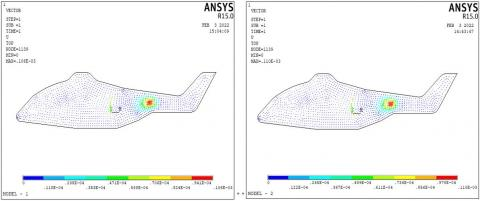## 2b.jpg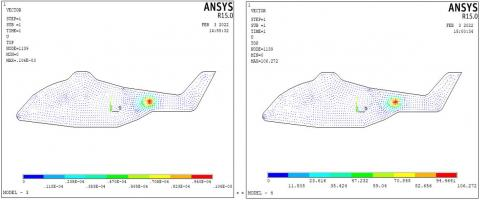## 2c.jpg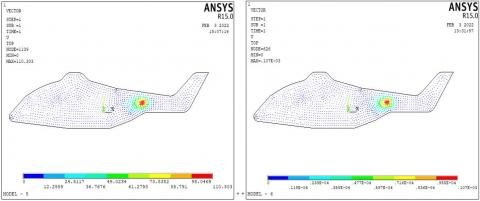Figure 2. Results of vector deformation (U) for all models

## 3a.jpg## 3b.jpg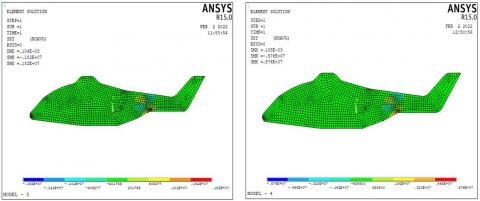## 3c.jpg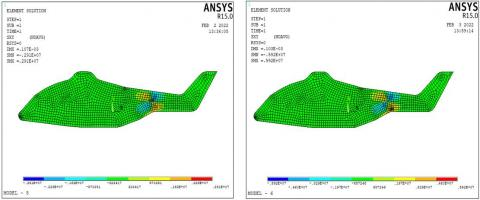Figure 3. Results of normal shear stress (бxy) for all models

Figure 6 shows the results obtained for the different models of strain intensity (Ԑint.), and the results were very close to each other and with a high degree of congruence, as the results were confined between (2.39*10-4 - 5.93*10-4).

## 4a.jpg## 4b.jpg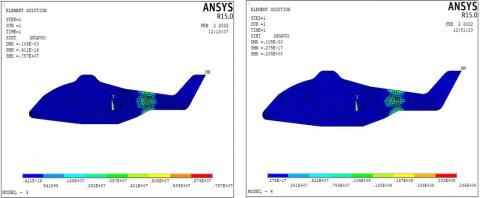## 4c.jpg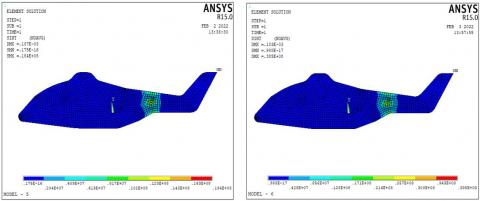Figure 4. Results of Normal intensity r stress (бint.) for all models

## 5a.jpg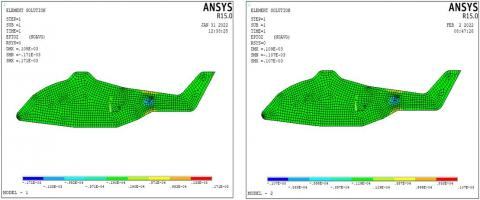## 5b.jpg## 5c.jpg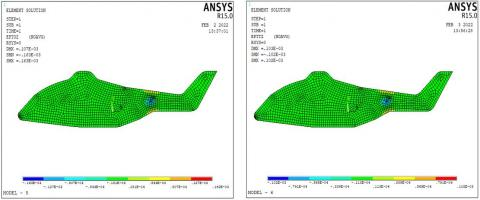Figure 5. Results of total mechanical strain (Ԑz) for all models

## 6a.jpg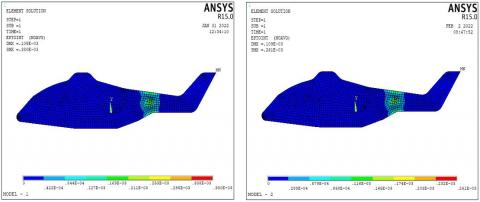## 6b.jpg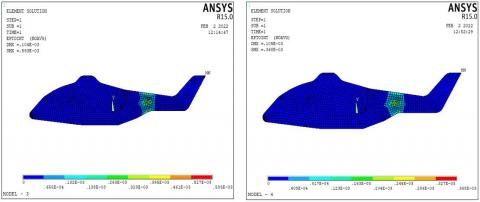## 6c.jpg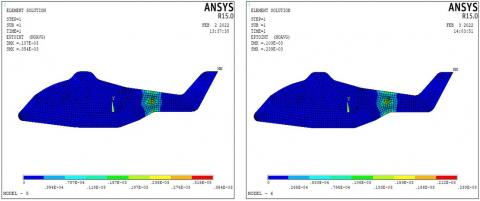Figure 6. Results of total mechanical strain intensity (Ԑint.) for all models

## 7a.jpg## 7b.jpg## 7c.jpgFigure 7. Results of body temperature (TB) for all models

Figure 7 shows the effect of load temperature on the helicopter structure for all models, and the figures show that the temperature distribution is similar in almost all models.

Figure 8 shows the geometry deformation (Uz) along the horizontal path from the beginning to the end and passing through the region of influence of the loads, and it shows in the figure the maximum deformation in the region of the influence of the load, which has a similar shape in all models.

## 8a.jpg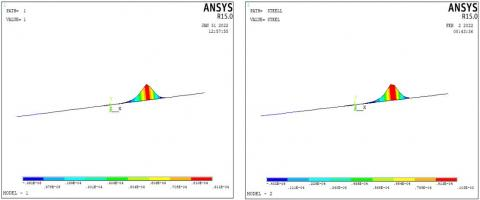## 8b.jpg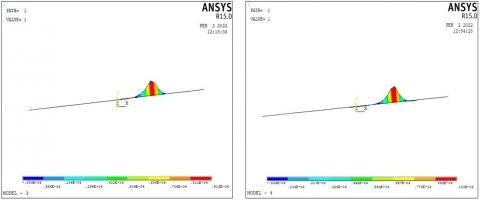## 8c.jpg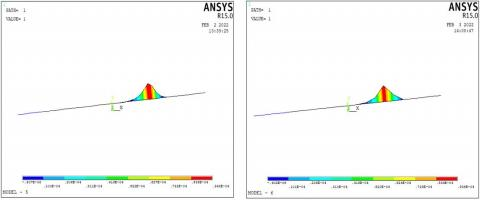Figure 8. Results of geometry deformation (Uz) on horizontal path for all models

Figure 9 appears the comparison of the results of deformation in the direction of (UZ) on the vertical path, and from the figure shows a match in the resistance of the different models, which are of different thicknesses.

## 9.pngFigure 9. Comparison showing the result of deformation (Uz) on the horizontal path for all models

Figure 10 appears a comparison of the results obtained for axial deformation in the direction of (ROTX) on the vertical path, and from the figure it is clear that the maximum deformation value in the first model is less than that of the other models.

## 10.png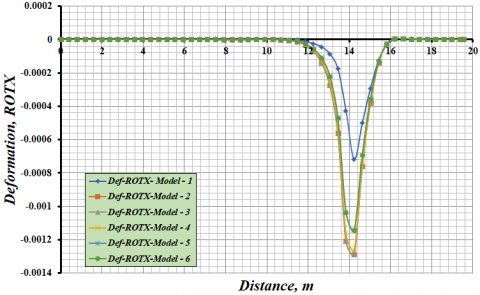Figure 10. Comparison showing the result of deformation (ROTX) on the horizontal path for all models

Figure 11 appears a comparison of the results obtained for axial deformation in the direction of (ROTY) on the vertical path, and the figure shows that the maximum deformation value is identical in all models.

## 11.png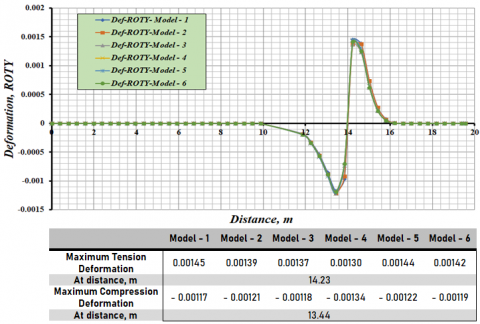Figure 11. Comparison showing the result of deformation (ROTY) on the horizontal path for all models

Figure 12 appears a comparison of the results obtained for axial deformation in the direction of (ROTSUM) on the horizontal path. The figure shows that the maximum deformation value was in the fifth model, and the deformation value was in the first mode, but the difference is very small between all models.

## 12.png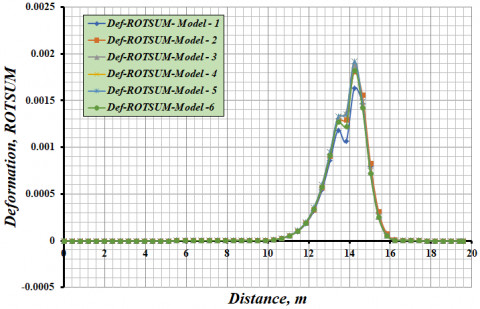Figure 12. Comparison showing the result of deformation (ROTSUM) on the horizontal path for all models

Figure 13 appears a comparison of the results obtained for normal stress (бx) on the horizontal path. The figure shows that the maximum value of the stress was in the sixth model and then the fifth model, while the lowest value of the stress was in the third model, also the stress value in the first model was almost in the average stresses.

## 13.png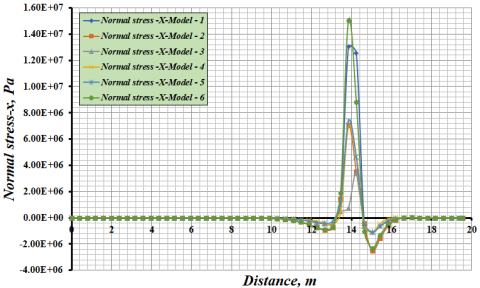Figure 13. Comparison showing the result of normal stress (бx) on the horizontal path for all models

Figure 14 appears a comparison of the results obtained for normal stress (бy) results on the horizontal path, and that the maximum value of the stress was in the second model, while it was the lowest value of the stress in the third model, and the stress value in the first model was after the value of second model.

## 14.png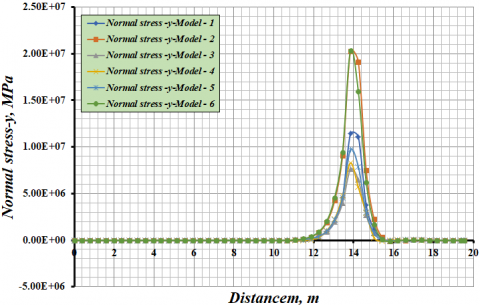Figure 14. Comparison showing the result of normal stress (бy) on the horizontal path for all models

Figure 15 appears a comparison of the results obtained for shear stress (τxy) on the horizontal path, and that the maximum value of shear stress was in the second model when it was positive shear stress, while it was the lowest value of the negative shear stress in the sixth model, and the value of the other models was almost equal.

## 15.pngFigure 15. Comparison showing the result of shear stress (τxy) on the horizontal path for all models

Figure 16 appears a comparison of the results obtained for the stress intensity (бint.) on the horizontal path, and that the maximum value of the stress intensity was in the second and sixth model, while it was the lowest value of the stress intensity in the third model, and the value of the other models was almost equal.

## 16.png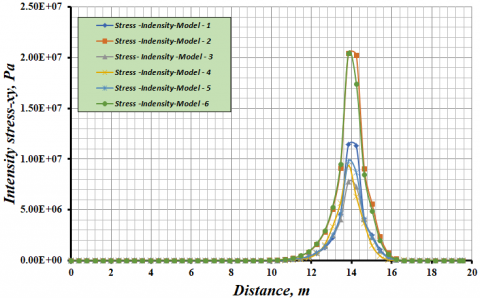Figure 16. Comparison showing the result of intensity stress (бint.) on the horizontal path for all models

Figure 17 appears a comparison of the results obtained of the stress results (бvon.) on the horizontal path, and that the maximum value of the stress intensity was in the second and sixth model, while the lowest value of the stress intensity was in the third model.

## 17.png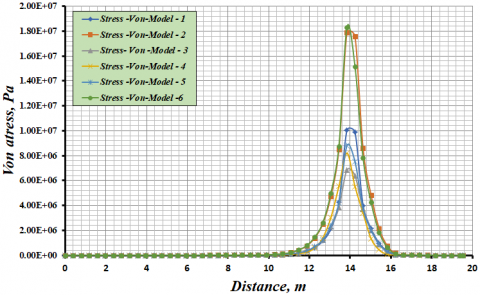Figure 17. Comparison showing the result of intensity stress (бvon.) on the horizontal path for all models

Figure 18 shows the comparison of the results of the total strain-x (Ԑx.) on the horizontal path, and an acceptable match is found in the results of all models.

## 18.png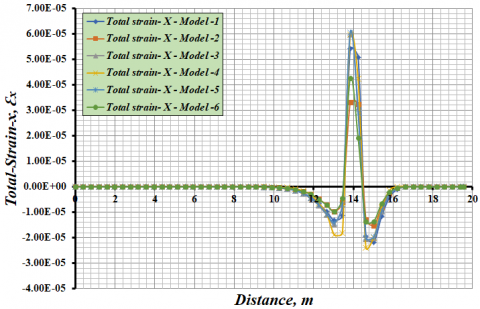Figure 18. Comparison showing the result of total strain-x (Ԑx.) on the horizontal path for all models

Figure 19 appears a comparison of the results obtained of the total strain (Ԑy) on the horizontal path, and the highest strains are in the first models compared to the other models, it was the lowest value in the sixth model.

## 19.pngFigure 19. Comparison showing the result of total strain-y (Ԑy.) on the horizontal path for all models

Figure 20 appears a comparison of the results obtained of the total strain (Ԑz) on the horizontal path, and the highest strains are in the first and fifth models compared to the other models, whose values are to some extent identical.

## 20.pngFigure 20. Comparison showing the result of total strain-z (Ԑz) on the horizontal path for all models

Figure 21 appears a comparison of the results obtained of the results of the shear total strain (Ԑxy) on the horizontal path, and the highest strains were in the fourth model compared to other models whose values were with an acceptable match.

## 21.png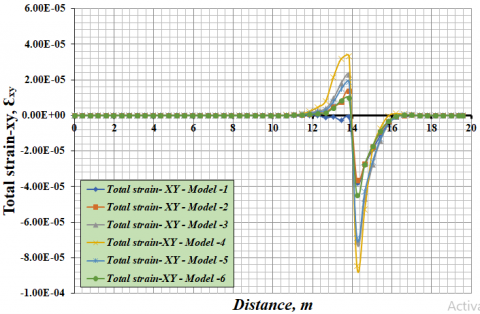Figure 21. Comparison showing the result of total strain-xy (Ԑx.y) on the horizontal path for all models

Figure 22 appears a comparison of the results obtained of the total strain intensity (Ԑint.) on the horizontal path, and the values of the second and sixth was the lowest, while the models' values were variable in the region of the highest strain, and the highest value was in the first model.

## 22.pngFigure 22. Comparison showing the result of total strain-intensity (Ԑint.) on the horizontal path for all models

Figure 23 appears a comparison of the results obtained of the total von strain (Ԑvon) on the horizontal path, where the lowest values were in the second and fifth models, while the other model values were somewhat identical.

## 23.png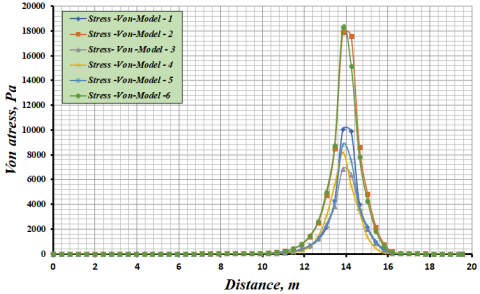Figure 23. Comparison showing the result of total von strain (Ԑvon) on the horizontal path for all models

Figure 24 appears a comparison of the results obtained of the axial deformation in the direction of (Uz) on the vertical path, and it is clear from the figure that the maximum deformation value in all models is similar.

## 24.png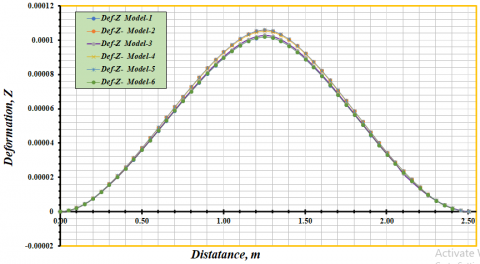Figure 24. Comparison showing the result of deformation (Uz) on the vertical path for all models

Figure 25 appears a comparison of the results obtained of the axial deformation in the direction of (ROTX) on the vertical path, and it is clear from the figure that the maximum deformation value in all models is similar.

## 25.png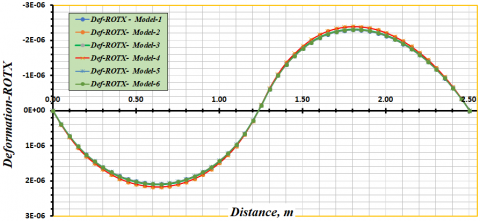Figure 25. Comparison showing the result of deformation (ROTX) on the vertical path for all models

Figure 26 appears a comparison of the results obtained of the axial deformation in the direction of (ROTY) on the vertical path, and the figure shows that the maximum deformation value is identical in all models.

## 26.png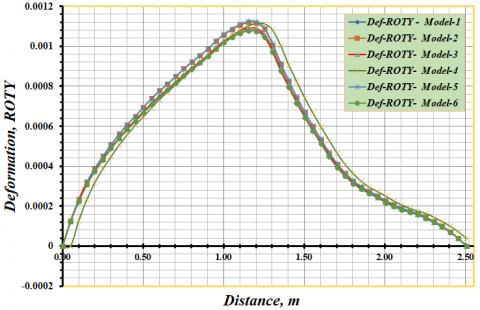Figure 26. Comparison showing the result of deformation (ROTY) on the vertical path for all models

Figure 27 appears a comparison of the results obtained of the axial deformation in the direction of (ROTSUM) on the vertical path. The figure shows nearly identical deformation values in all six different models.

## 27.png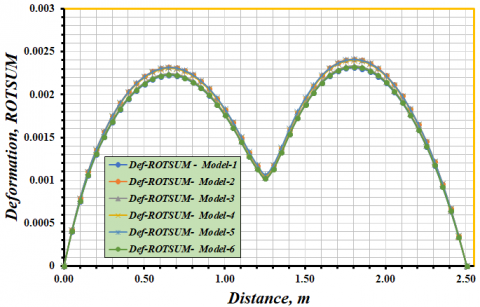Figure 27. Comparison showing the result of deformation (ROTSUM) on the vertical path for all models

Figure 28 appears a comparison of the results obtained of the normal stress (бx) on the vertical path. The figure shows that the maximum value of the stress was in the second model, while the lowest value of the stress was in the third model.

## 28.png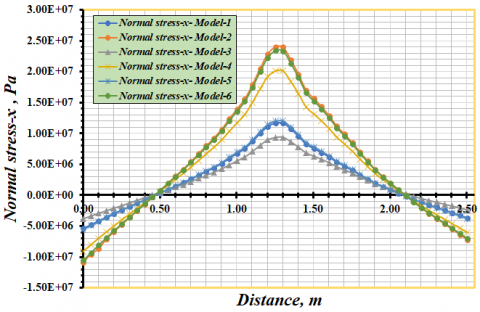Figure 28. Comparison showing the result of normal stress (бx) on the vertical path for all models

Figure 29 appears a comparison of the results obtained of the normal stress (бy) results on the vertical path, and that the maximum value of the stress was in the second model, while it was the lowest value of the stress in the third model.

## 29.pngFigure 29. Comparison showing the result of normal stress (бy) on the vertical path for all models

Figure 30 appears a comparison of the results obtained of the shear stress results (τxy) on the vertical path, and that the maximum value of shear stress was in the second model when it was positive shear stress, while it was the lowest value of the negative shear stress in the first model, and the value of the other models was almost equal.

## 30.pngFigure 30. Comparison showing the result of normal stress (τxy) on the vertical path for all models

Figure 31 appears a comparison of the results obtained of the stress intensity (бint.) on the vertical path, and that the maximum value of the stress intensity was in the second and sixth model, while it was the lowest value of the stress intensity in the third model.

## 31.png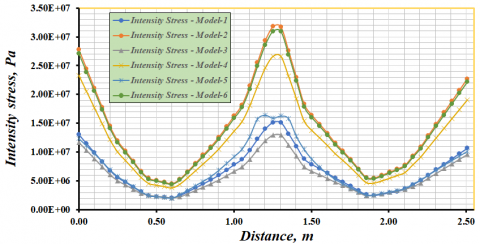Figure 31. Comparison showing the result of intensity stress (бint.) on the vertical path for all models

Figure 32 appears a comparison of the results obtained of the von stress results (бvon.) on the vertical path, and that the maximum value of the von stress was in the second model, while the lowest value of the stress intensity was in the third model.

## 32.png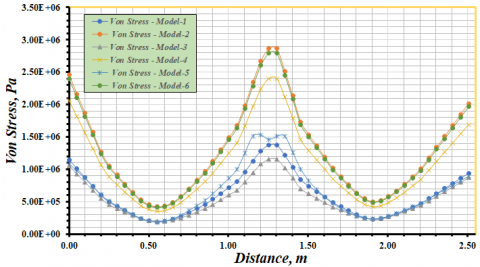Figure 32. Comparison showing the result of intensity stress (бvon.) on the vertical path for all models

Figure 33 appears a comparison of the results obtained of the total strain-x (Ԑx) on the vertical path, and the strain was highest in the fourth model and lowest in the sixth model.

## 33.pngFigure 33. Comparison showing the result of total strain-x (Ԑx.) on the vertical path for all models

Figure 34 appears a comparison of the results obtained of the total strain (Ԑy) on the vertical path, and the highest strains are in the first models compared to the other models, it was the lowest value in the sixth model.

## 34.png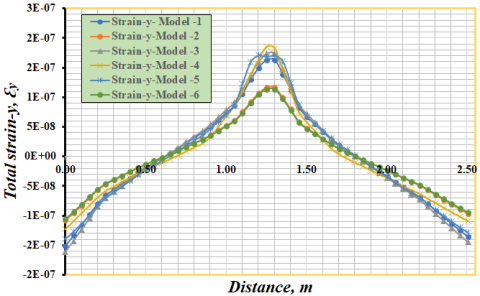Figure 34. Comparison showing the result of total strain-y (Ԑy.) on the vertical path for all models

Figure 35 appears a comparison of the results obtained of the total strain (Ԑz) on the vertical path, and the highest strains are in the first and fifth models compared to the other models, whose values are to some extent identical.

## 35.pngFigure 35. Comparison showing the result of total strain-z (Ԑz) on the vertical path for all models

Figure 36 appears a comparison of the results obtained of the shear total strain (Ԑxy) on the vertical path, and the highest strains were in the third model compared to other models whose values were with an acceptable match.

## 36.pngFigure 36. Comparison showing the result of total strain-xy (Ԑxy) on the vertical path for all models

Figure 37 appears a comparison of the results obtained of the total strain intensity (бint.) on the vertical path, and the value of the sixth was the lowest, and the highest value was in the second model.

## 37.png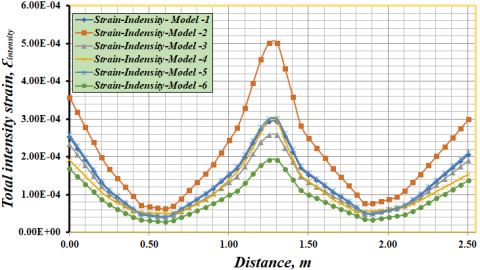Figure 37. Comparison showing the result of total strain-intensity (Ԑint.) on the vertical path for all models

Figure 38 appears a comparison of the results obtained of the total von strain (Ԑvon) on the vertical path, where the lowest values were in the second and sixth models, while largest value in the fourth model.

## 38.pngFigure 38. Comparison showing the result of total von strain (Ԑvon) on the vertical path for all models

Table 4 shows the results of all the tests for the six models that were designed in the ANSYS program to obtain better specifications for the wall of the structure of a helicopter.

The results in Table 4 show the following:

1. The weight of one square meter of the helicopter made of aluminum is (81 kg), as the lowest weight of the helicopter was when using carbon fiber materials, as the weight of one square meter was (54.51 kg) and thickness (28 mm), which is a decrease about aluminum by (22.7 %) and this is very better in terms of weight, followed by a wall consisting of an outer layer thick (5 mm) and an inner layer (5 mm) of aluminum and a middle layer of carbon fiber (18 mm thick), where the weight of the wall was per square meter (64 .98 mm), that is, less than a wall of aluminum by (19.2 %). It was higher and significantly higher than aluminum for a steel wall, where the weight was (156 kg) for one square meter of steel and (20 mm) thick, Where the percentage increase over the weight of aluminum by 93%.
2. The results indicate that the values of maximum deformation shape, total mechanical strain (Ԑz) and total mechanical strain intensity (Ԑint.) are in an acceptable match.
3. The results in the above table indicate that the highest value of normal stress was in the sixth model (33 MPa), and the lowest value was in the third model (7.28 MPa), while the results of shear stress show that the highest value was in the fourth model (7.78 MPa) and the lowest in the third model (1.82 MPa). Also the results of stress intensity indicate that the highest value of the stress intensity was in the second model (42.57 MPa), while it was the lowest value in the third model (7.57 MPa), and these results of stresses show that the resistance of the third model is the weakest, While the optimum stress strength in the second model is very close to the values of the different stresses in the sixth model.

Table 4. The results of the six models tests using a ANSYS 15.0 program

 Model Materials Density, ρ, g/m3 Deformation shape, Maximum Normal stress stress-x, MPa Shear stress stress-xy, MPa Intensity stress, MPa Total mechanical strain -z Total mechanical strain intensity Number of layers Total thickness, t, (mm) Volume of wall, m3 Weight, of one square meter, (Kg) Percentage of the weight of a square meter of material compared to the weight of a square meter of aluminum % M1 Aluminum Alloy 6061 - T6 2700 1.05*10-4 16.1 2.85 19.7 1.73*10-4 3.8*10-4 1 30 0.03 81 --- M2 Steel 7800 1.09*10-4 33 6.8 42.1 1.07*10-4 4.21*10-4 1 20 0.02 156 1.93 M3 Fiberglass Glass70% 2500 1.04*10-4 7.28 1.82 7.57 3.23*10-4 7.57*10-4 45 45 0.045 94.95 1.17 Epoxy30% 1200 M4 Carbon fiber Carbon70% 2267 1.07*10-4 21 7.76 23.5 1.33*10-4 3.68*10-4 28 28 0.028 54.51 0.673 Epoxy30% 1200 M5 AA - 6061 - T6 & Carbon fiber AA - 6061 2700 1.07*10-4 15.9 2.91 18.4 1.63*10-4 3.54*10-4 2 10 0.01 64.98 0.802 Carbon70% 2500 18 18 0.018 Epoxy30% 1200 M6 Steel & Carbon fiber Steel 7800 1.04*10-4 33.3 6.02 39.4 1.03*10-4 2.43*10-4 2 10 0.01 101.21 1.25 Carbon70% 2500 11 11 0.011 Epoxy30% 1200
4. Conclusions

The values of deformations, stresses, strains and temperature distribution were extracted in a three-dimensional model consisting of aluminum alloy (AA-6061- T6), and then five other models were designed with values of deformation resistance and resistance to stresses and strains close to the first model, but with different thicknesses to obtain the best structure with the least weight, The following conclusions were obtained:

1. The results show that the best weight obtained from a square of the structure of the helicopter was in the fourth model consisting of carbon fiber, where the weight was (54.41 kg ) and the wall thickness was (28 mm), and then the fifth model, which consisted of aluminum alloy from the inside and outside with two layers, and between them carbon fiber with eighteen layers where the weight was (64.98 kg), but the worst model that had a large weight was the second model made of steel where the weight was (156 kg), despite the fact that its thickness was less compared to the other models, and the reason for this is its high density.
2. The values of resistance to deformations as shown by the figures in all results and in all directions (UX, UY, UZ, ROTX, ROTY, ROTZ, ROTSUM) were almost equal, meaning that the percentages of difference were very small.
3. There was a discrepancy in the values of all the different stresses (бx, бy, бz, τxy, τyz, τxz, бint., бvon) on the helicopter structure, in general, the maximum stresses were in the second model consisting of steel, and the lowest in the third model consisting of fiberglass, while the different stresses resistance values for the other models were between the values of the second and third models and the differences were between them in a rather small proportion.
4. The percentage of variance in the different strain resistance values (Ԑx, Ԑy, Ԑz, Ԑxy, Ԑyz, Ԑxz, Ԑint., Ԑvon) in all models was very small, and it can be said that the results, as shown in the figures for strain resistance, indicate that the strains are equal in all models.
5. The effect of high load temperature and its distribution on the surface of the helicopter's structure, as shown in the figures for the effect of temperature in the loading area on the helicopter's structure, was almost the same, as the difference ratios were very few in all models.
Acknowledgment

This research was supported by Engineering Science Research Program through the Northern Technical University / Technical Engineering college and Technical Institute of Mosul funded by the Ministry of Higher Education and Scientific Research / Republic of Iraq. (Grant No.: 00218-2021).

References

 Thangaratnam, R.K., Ramachandran, J. (1988). Thermal stress analysis of laminated composite plates and shells. Computers & Structures, 30(6): 1403-1411. https://doi.org/10.1016/0045-7949(88)90204-0

 Abrate, S. (2001). Modeling of impacts on composite structures. Composite Structures, 51(2): 129-138. https://doi.org/10.1016/S0263-8223(00)00138-0

 Hogg, P.J. (2003). Composites for ballistic applications. Proceedings of Composite Processing, pp. 1-11. https://doi.org/10.1126/science.1131118

 Leong, M., Sankar, B.V. (2010). Effect of thermal stresses on the failure criteria of fiber composites. Mechanics of Advanced Materials and Structures, 17(7): 553-560. https://doi.org/10.1080/15376490903398706

 Vemuri, M., Bhat, B.T. (2011). Armour protection and affordable protection for futuristic combat vehicles. Defence Scientific Journal, 61: 394-402. https://doi.org/10.14429/dsj.61.365

 Hedayati, R., Ziaei-Rad, S., Eyvazian, A., Hamouda, A. M. (2014). Bird strike analysis on a typical helicopter windshield with different lay-ups. Journal of Mechanical Science and Technology, 28(4): 1381-1392. https://doi.org/10.1007/s12206-014-0125-3

 Cimpoeru, S.J. (2016). The mechanical metallurgy of armour steels. Defence Science and Technology Group Fishermans Bend VIC Australia. https://www.dst.defence.gov.au/sites/default/files/publications/documents/DST-Group-TR-3305_0.pdf.

 Vu, N.A., Lee, J.W., Le, T.P.N., Nguyen, S.T.T. (2016). A fully automated framework for helicopter rotor blades design and analysis including aerodynamics, structure, and manufacturing. Chinese Journal of Aeronautics, 29(6): 1602-1617. https://doi.org/10.1016/j.cja.2016.10.001

 Schwinn, D., Weiand, P., Schmid, M. (2017). Structural analysis of a rotorcraft fuselage in a multidisciplinary environment. In Summary of Proceedings. https://elib.dlr.de/112814.

 Schwinn, D.B., Weiand, P., Buchwald, M. (2020). Structural sizing of a rotorcraft fuselage using an integrated design approach. Journal of the American Helicopter Society, 65(4): 1-12. https://doi.org/10.4050/JAHS.65.042008

 Brown, C.D., Series, A.E. (1992). American Institute of Aeronautics and Astronautics, 370 L’Engant Promenade. SW, Washinton DC. https://arc.aiaa.org/doi/book/10.2514/4.104909

 Schwinn, D. B., Weiand, P., Buchwald, M. (2021). Rotorcraft fuselage mass assessment in early design stages. CEAS Aeronautical Journal, 12(2): 307-329. https://doi.org/10.1007/s13272-021-00492-z

 Reddy, J.N., Hsu, Y.S. (1980). Effects of shear deformation and anisotropy on the thermal bending of layered composite plates. Journal of Thermal Stresses, 3(4): 475-493. https://doi.org/10.1080/01495738008926984

 Khdeir, A.A., Reddy, J.N. (1991). Thermal stresses and deflections of cross-ply laminated plates using refined plate theories. Journal of Thermal Stresses, 14(4): 419-438. https://doi.org/10.1080/01495739108927077

 Kant, T., Khare, R.K. (1994). Finite element thermal stress analysis of composite laminates using a higher-order theory. Journal of Thermal Stresses, 17(2): 229-255. https://doi.org/10.1080/01495739408946257

 Robaldo, A. (2006). Finite element analysis of the influence of temperature profile on thermoelasticity of multilayered plates. Computers & Structures, 84(19-20): 1236-1246. https://doi.org/10.1016/j.compstruc.2006.01.022

 Cho, M., Oh, J. (2003). Higher order zig-zag plate theory under thermo-electric-mechanical loads combined. Composites Part B: Engineering, 34(1): 67-82. https://doi.org/10.1016/J.IJSOLSTR.2006.04.017

 Zhen, W., Wanji, C. (2009). A higher-order displacement model for stress concentration problems in general lamination configurations. Materials & Design, 30(5): 1458-1467. https://doi.org/10.1016/j.matdes.2008.08.013

 Sayyad, A.S., Ghugal, Y.M., Shinde, B.M. (2016). Thermal stress analysis of laminated composite plates using exponential shear deformation theory. International Journal of Automotive Composites, 2(1): 23-40. https://doi.org/10.1504/IJAUTOC.2016.078100

 Noor, A.K., Burton, W.S. (1992). Computational models for high-temperature multilayered composite plates and shells. Applied Mechanics Reviews, 45(10): 419-446. https://doi.org/10.1115/1.3119742

 Argyris, J., Tenek, L. (1997). Recent advances in computational thermostructural analysis of composite plates and shells with strong nonlinearities. Applied Mechanics Reviews, 50: 285-306. https://doi.org/10.1115/1.3101708

 Carrera, E. (2002). Temperature profile influence on layered plates response considering classical and advanced theories. AIAA Journal, 40(9): 1885-1896. https://doi.org/10.2514/2.1868

 Karash, E.T., Alsttar Sediqer, T.A., Elias Kassim, M.T. (2021). A comparison between a solid block made of concrete and others made of different composite materials. Revue des Composites et des Matériaux Avancés, 31(6): 341-347. https://doi.org/10.18280/RCMA.310605

 Najim, M., Sultan, J., Karash, E. (2020). Comparison of the resistance of soild shell of composite materials with other soild metal Materials. In: IMDC-SDSP 2020, pp. 28-30. https://doi.org/10.4108/eai.28-6-2020.2298518

 Karash, E.T. (2011). Modelling of unilateral contact of metal and fiberglass shells. In Applied Mechanics and Materials. 87: 206-208. https://doi.org/10.4028/www.scientific.net/AMM.87.206

 Cho, K.N., Striz, A.G., Bert, C.W. (1989). Thermal stress analysis of laminate using higher-order theory in each layer. Journal of Thermal Stresses, 12(3): 321-332. https://doi.org/10.2514/6.1989-1223

 Zhang, Z., Zhou, D., Fang, H., Zhang, J., Li, X. (2021). Analysis of layered rectangular plates under thermo-mechanical loads considering temperature-dependent material properties. Applied Mathematical Modelling, 92: 244-260. https://doi.org/10.1016/j.apm.2020.10.036

 Wu, Z., Chen, W, Ren, X. (2007). Refined global-local higher-order theory and finite element for laminated plates. International Journal for Numerical Methods in Engineering, 69(8): 1627-1670. https://doi.org/10.1016/j.compstruct.2008.06.011